# Characteristics of Beams for Its Analysis and DesignReading time: 1 minute

There are a number of properties of a beam that an Engineer should be aware of as they dictate beam behaviour when subject to a load and ultimately represent possible areas or mechanisms for failure. The main ones being:
• Second moment of area (also referred to as the second moment of inertia): this depends on the cross section profile of the beam and is a measure of the resistance of the shape of the beam to bending.
• Bending moment: usually illustrated on a bending moment diagram, and often related the deflection of the beam, can be used to calculate regions subject to maximum bending forces and consequently most likely to yield. It also illustrates which sections of the beam are in compression or tension.
• Beam deflection: beam deflection tends to be undesirable and correlates to the bending moment.
• Shear diagrams: these are used to illustrate stress concentrations along the beam and provide a means to identify areas of maximum shear forces where the beam is more likely to fail by shear.
>Second moment of area The second moment of area (I) is a property of the shape used to predict the resistance of the beam to bending and deflection. It is calculated from the physical cross sectional area of the beam and relates the profile mass to the neutral axis (this being a region where the beam is subject to neither compression or tension, as labelled in figure 5. It is dependant on the direction of loading; for most beams except both hollow and solid box and circular sections, the second moment of area will be different when loaded from a horizontal or vertical direction.Figure 5 – a) simply supported beam of length l with no force; b) simply supported beam subject to point load (force) F at centre creating bending. The second moment of area can be calculated from first principles for any cross section profile using the equation: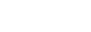However, for common beam profiles standard formula are used: I – beam / Universal beamFigure 6 – I-beam cross-section profile with loading parallel to web. The I – beam or Universal beam has the most efficient cross sectional profile as most of its material is located away from the neutral axis providing a high second moment of area, which in turn increases the stiffness, hence resistance to bending and deflection. It can be calculated using the formula: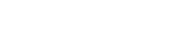As shown in figure 6, this is only suitable for loading parallel to the web, as loading perpendicular to the web would be less efficient. Box sectionFigure 7 – Box section cross-section profile

The box section has the most efficient profile in loading both horizontally and vertically. It has a lower value for second moment of area so is less stiff. It can be calculated by using the formula: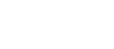## Bending moment and shear diagrams

Bending moment and shear diagrams are typical drawn alongside a diagram of the beam profile as shown below, this enables an accurate representation of the beams behaviour. a) represents a beam subject to a uniformly distributed load (udl) of magnitude w, across its length, l. Total force on beam being wl. The beam is simply supported with reaction forces R. Distance x represents any point along the beam. b) shear force diagram shows the regions of maximum shear, for this beam these correlate to the reaction forces. The slope of the shear force diagram is equal to the magnitude of the distributed load. A positive shear force will cause the beam to rotate clockwise and a negative shear force will cause the beam to rotate in an anticlockwise direction. c) maximum bending moment occurs when no shear forces exist on the beam. As the beam is simply supported, that is only subject to vertical reaction forces, no bending moment is experienced at these points. If the beam were restricted as in a cantilever situation then bending moments would be experienced at either end Correlating to the diagrams of beam loading, shear force and bending moments maximums and values at distance x along the beam can be calculated using the following formula: Reaction force and maximum shear force and Shear force at distance x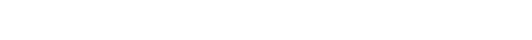Maximum bending moment and Bending moment at distance x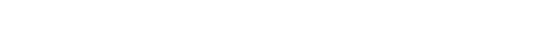Maximum deflection and Deflection at distance xThese formulas are specific to this beam situation, that is a uniformly distributed load with simple supports as shown. For a cantilever beam, or one with varying degrees of freedom at the supports (this refers to restrains in the horizontal direction subjecting the beam to a turning moment at this location) then different formula will be required. All formula can be calculated from first principles but for convenience look-up-tables such as those contained in “Roark’s formulas for stress and strain” can be utilised. The equations for maximum beam deflection, and deflection at distance x are shown to be dependant on the Young’s modulus, E and second moment of area, I, where as shear force and bending moment are independent of these beam characteristics.